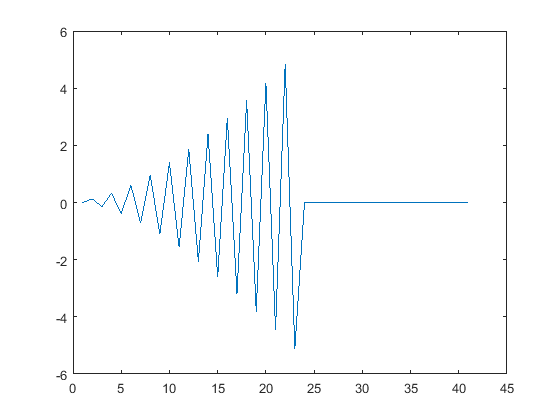# 7.2: Flowcharts

•• Carey Smith
• Oxnard College
$$\newcommand{\vecs}{\overset { \rightharpoonup} {\mathbf{#1}} }$$ $$\newcommand{\vecd}{\overset{-\!-\!\rightharpoonup}{\vphantom{a}\smash {#1}}}$$$$\newcommand{\id}{\mathrm{id}}$$ $$\newcommand{\Span}{\mathrm{span}}$$ $$\newcommand{\kernel}{\mathrm{null}\,}$$ $$\newcommand{\range}{\mathrm{range}\,}$$ $$\newcommand{\RealPart}{\mathrm{Re}}$$ $$\newcommand{\ImaginaryPart}{\mathrm{Im}}$$ $$\newcommand{\Argument}{\mathrm{Arg}}$$ $$\newcommand{\norm}{\| #1 \|}$$ $$\newcommand{\inner}{\langle #1, #2 \rangle}$$ $$\newcommand{\Span}{\mathrm{span}}$$ $$\newcommand{\id}{\mathrm{id}}$$ $$\newcommand{\Span}{\mathrm{span}}$$ $$\newcommand{\kernel}{\mathrm{null}\,}$$ $$\newcommand{\range}{\mathrm{range}\,}$$ $$\newcommand{\RealPart}{\mathrm{Re}}$$ $$\newcommand{\ImaginaryPart}{\mathrm{Im}}$$ $$\newcommand{\Argument}{\mathrm{Arg}}$$ $$\newcommand{\norm}{\| #1 \|}$$ $$\newcommand{\inner}{\langle #1, #2 \rangle}$$ $$\newcommand{\Span}{\mathrm{span}}$$$$\newcommand{\AA}{\unicode[.8,0]{x212B}}$$

By Carey A. Smith

A flowchart is a graphical representation of code logic. It is especially helpful to understand the logic of code with for loops and if-else logic.

https://en.wikipedia.org/wiki/Flowchart [en.Wikipedia.org]

##### Example $$\PageIndex{1}$$ Odd-Even Flow ChartTry to code this up
Solution

The corresponding code is:

for n = 1:12     if(mod(n,2) == 0)         disp([num2str(n),' is even'])     else         disp([num2str(n),' is odd'])     end end

.

##### Example $$\PageIndex{2}$$ flowchart for primes

This flowchart and code show an algorithm to determine which numbers between 1 and 48 are prime numbers.

We already know that 2, 3, and 5 are prime, so the for loop starts at k = 6.

For each value it tests, it displays a message stating whether or not it is a prime number. It stores the prime numbers it finds in a vector called prime_list.Try coding this logic.

Note that the for-loop automatically does the "k = k+1" shown at the bottom of the flowchart; do not code that line.

(There are more sophisticated and efficient prime-number algorithms; this one is an illustration of moderately complex logic.)

###### Solution

%% "for loop" example with “if-then-elseif” logic: % Find the prime numbers between 1 to 48: prime_list = [2,3,5] % Initial set of primes for k = 6:48     if( mod(k,2) == 0) % Tests if k is divisible by 2         disp([num2str(k),' is not prime. It is divisible by 2'])     elseif (mod(k,3) == 0) % Tests if k is divisible by 3         disp([num2str(k),' is not prime. It is divisible by 3'])     elseif( mod(k,5) == 0) % Tests if k is divisible by 5         disp([num2str(k),' is not prime. It is divisible by 5'])     else         disp([num2str(k),' is prime.'])         % Add k to the list of primes:         prime_list = [prime_list, k];     end % end if end % for k = 6:48 % Display the primes found prime_list

This code is the attached file for_loop_primes.m

.

##### Example $$\PageIndex{3}$$ Divergent Series1

This is an example of a divergent series. Instead of the terms getting smaller, these terms get larger in absolute value.

In this example the line continuation symbol of 3 dots is used to continue the code on the next line.

clear all; close all; format compact; clc;
n = 40; % maximum number of iterations total = zeros(1,(n+1)); term_lim = 10; % Terminate the sequence if termk > term_lim for k = 2:n     termk = (-1)^k*(k^2)/(k+30);     if( abs(termk) > term_lim)         disp(['k= ',num2str(k),...             ', termk = ',num2str(termk),...             '>10, so break the loop'])         break;     else         total(k) = total(k-1) + termk;     end end figure; plot(total)

###### Solution.

## Homework:

1. Watch the following video from Robert Talbert

Then code the second example in the video about the temperature and rain. The corresponding flowchart is this:Figure $$\PageIndex{i}$$: Flowchart for Temperature, Rain, Wearing a Coat.

Put parentheses around the conditional expressions, even though the video did not do this. Examples:

if (temp < 50)

elseif (raining == 1)

.

##### Exercise $$\PageIndex{1}$$ Divergent Series--Car Loss of Control

Read Matlab's description of the "break" keyword at this link: break keyword (Links to an external site.)(opens in new window)

% Divergent loop due to unstable feedback
% Car 1 (in freeway lane 1 next to the center divider) passes a truck in lane 2.
% Car 1 begins to enter lane 2.
% But car 1's driver sees car 3 in lane 3 (on the right) also start to change lanes into lane 2.
% So car 1's driver turns hard to the left.
% Due to the speed of the car and the delay to realize how far he has turned, each time he "over corrects".
% Set
r = 2.1 % Over correction factor
% Model this with the following, where x is the number of feet he has moved to the right.
% (Negative values are to the left.)

% Before the for loop, initial the vector of position vs. time with this code:

m = 1; x(m) = 3; % position when he make his 1st correction

% Write a for loop with index m:

for m = 2:20

    In the for loop, include the following steps:     % The change in position each iteration is     dx = -r*x(m-1);     % Add this to the current position:     x(m) = x(m-1) + dx;     % Use a compound if-statement such that if 

    %   either the absolute value of dx is greater than 15,     %   or the absolute value of x(m) is greater than 10,     %   then use the "break" keyword to stop looping.

end

% After the for-loop, open a new figure and plot x. figure; plot(x) % Turn on the grid grid on % Add this title: title('Divergent car control loop')

% After running the code, check the value of the index, m. If m is >13, then the loop did not terminate when it should have. In this case, check the code to verify that the conditional statement used abs(dx) and abs(x(m)).